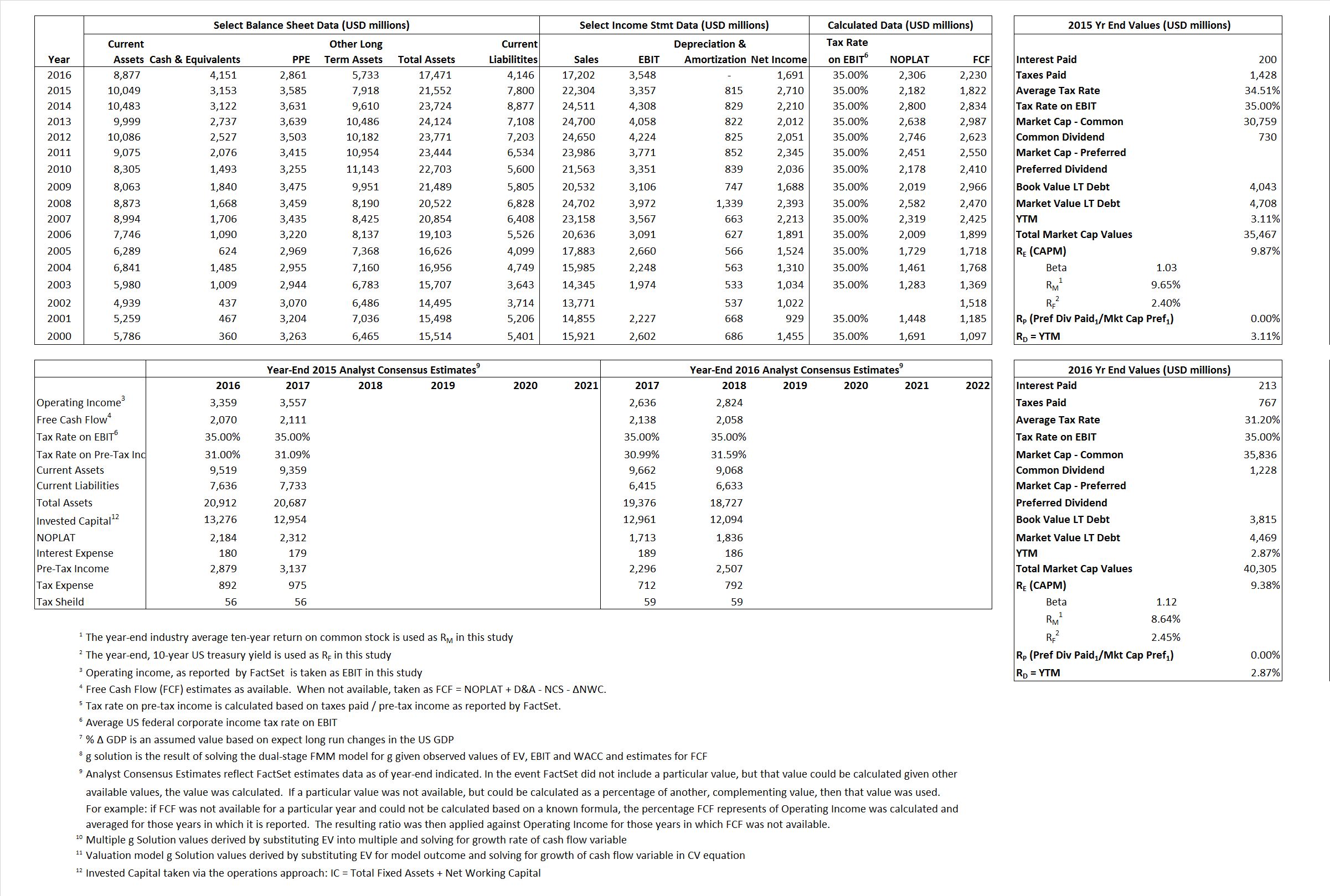# Emerson Eletric Co.

## Analyst Listing

The following analysts provide coverage for the subject firm as of May 2016:

 Broker Analyst Analyst Email Langenberg, LLC Brian K. Langenberg brian@langenberg-llc.com Oppenheimer Christopher glynn christopher.glynn@opco.com RBC Capital Markets Deane M. Dray deane.dray@rbccm.com Longbow Research Eli Lustgarten elustgarten@longbowresearch.com Cowen & Company Gautam Khanna gautam.khanna@cowen.com Deutsche Bank Research john g. Inch john.inch@db.com CRT Capital Group Josh W. Sullivan jwsullivan@sterneageecrt.com Buckingham Research Joshua Pokrzywinski jpokrzywinski@buckresearch.com Credit Suisse Julian Mitchell julian.mitchell@credit-suisse.com William Blair Nicholas P. Heymann nheymann@williamblair.com Wells Fargo Securities Richard M. Kwas rich.kwas@wellsfargo.com Atlantic Equities Richard Radbourne r.radbourne@atlantic-equities.com Stifel Nicolaus Robert P. McCarthy mccarthyr@stifel.com Bernstein Research Steven E. winoker steven.winoker@bernstein.com

## Primary Input Data## Derived Input Data

### Equational Form

Net Operating Profit Less Adjusted Taxes NOPLAT  2,182  2,306$NOPLAT\, =\, EBIT\, x\, (1 \,-\, Avg \,\,Tax\,\, Rate\,\, on\,\, EBIT)$
Free Cash Flow FCF 1,822 2,230$FCF\,=NOPLAT\,+\,Non-Cash\,Expenses-\Delta NWC\,-\,NCS$
Tax Shield TS 69 66$TS\,=\,Interest\,\,Paid\,\,x\,\, Avg \,\,Tax\,\,Rate\,\, on\,\, Pre-Tax\,\, Income$
Invested Capital IC 13,752 13,325$IC\,=\,Fixed\,\,Operating\,\,Assets\,\,+\,\,Net\,\, Working\,\, Capital$
Return on Invested Capital ROIC 15.87% 17.31%$ROIC\,=\,\frac { NOPLAT }{ IC }$
Net Investment NetInv (280)  (427)$NetInv\,=\,{ {IC}_{1}}-{{IC}_{0}}+Depreciation$
Investment Rate IR -12.83% -18.52%$IR\,=\,\frac {NetInv}{NOPLAT}$
Weighted Average Cost of Capital
WACCMarket 8.83%  8.56%$WACC\,=\,\frac { E }{ V } { R }_{ E }\,+\,\frac { P }{ V } { R }_{ P }\,+\,\frac { D }{ V } { R }_{ D }\left( 1- Avg\,\, Tax\,\,Rate\,\,on\,\,Pre-Tax\,\,Income \right)$
WACCBook  8.62%  9.09%
Enterprise value
EVMarket 32,314  36,154$EV\,=\,Market\,\,Cap\,\,Equity\,+\,\,Long\,\,Term\,\,Debt\,-\,Cash$
EVBook  31,649  35,500
Long-Run Growth
g = IR x ROIC
-2.04%  -3.20% Long-run growth rates of the income variable are used in the Continuing Value portion of the valuation models.
g = %$\Delta$ GDP    2.50%   2.50%
Margin from Operations M  15.05%  20.63%$M\,\,=\,\,\frac{EBIT}{SALES}$
Depreciation/Amortization Rate D  19.53%   0.00%$D\,\,=\,\,\frac{D+A}{EBITDA}$

## Valuation Multiple Outcomes

The outcomes presented in this study are the result of original input data, derived data, and synthesized inputs.

### model g solution

12/31/2015 12/31/2016 12/31/2015 12/31/2016 12/31/2015 12/31/2016

EV/SALES$\frac {EV}{Sales} \,= \,\frac{ROIC\, -\, g}{ROIC\,(WACC\,-\,g)}\,(1\,-\,T)\,(M)$

1.45  2.10  3.61%  3.46%  4.03%  4.58%

EV/EBITDA$\frac {EV}{EBITDA} \,= \,\frac{ROIC\, -\, g}{ROIC\,(WACC\,-\,g)}\,(1\,-\,T)\,(1\,-\,D)$

7.75 10.19 3.61% 3.46% 4.03% 4.58%

EV/NOPLAT$\frac {EV}{NOPLAT} \,= \,\frac{ROIC\, -\, g}{ROIC\,(WACC\,-\,g)}$

14.81 15.68 3.61% 3.46% 4.03% 4.58%

EV/FCFOPS$\frac {EV}{FCF_{OPS}} \,= \,\frac{ROIC\, -\, g}{ROIC\,(WACC\,-\,g)}\,(1\,-\,T)$

17.74 16.21 3.61% 3.46% 4.03% 4.58%

EV/EBIT$\frac {EV}{EBIT} \,= \,\frac{ROIC\, -\, g}{ROIC\,(WACC\,-\,g)}\,(1\,-\,T)$

9.63 10.19 3.61% 3.46% 4.03% 4.58%

EV/IC$\frac {EV}{IC} \,= \,\frac{ROIC\, -\, g}{WACC\,-\,g}$

2.35 2.71 3.61% 3.46% 4.03% 4.58%